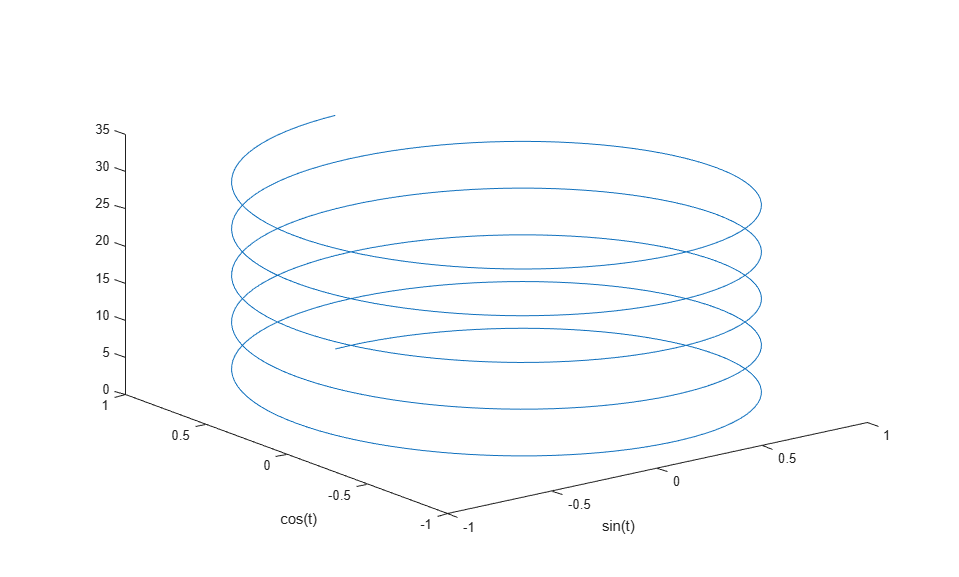Creating 3-D Plots

This example shows how to create 3-D line plots in MATLAB using the plot3 function.

Create a regularly-spaced vector t from 0 to 10*pi using pi/50 as the increment between elements.

t = 0:pi/50:10*pi;

Calculate sine for each value in t.

st = sin(t);

Calculate cosine for each value in t.

ct = cos(t);

Use the 'plot3' function to display a three-dimensional plot of a set of data points.

figure;
plot3(st, ct, t)
xlabel('sin(t)')
ylabel('cos(t)')